# use LFSR to encrypt and decrypt some message, and use two or more different structure of LFSR.

use LFSR to encrypt and decrypt some message, and use two or more different structure of LFSR.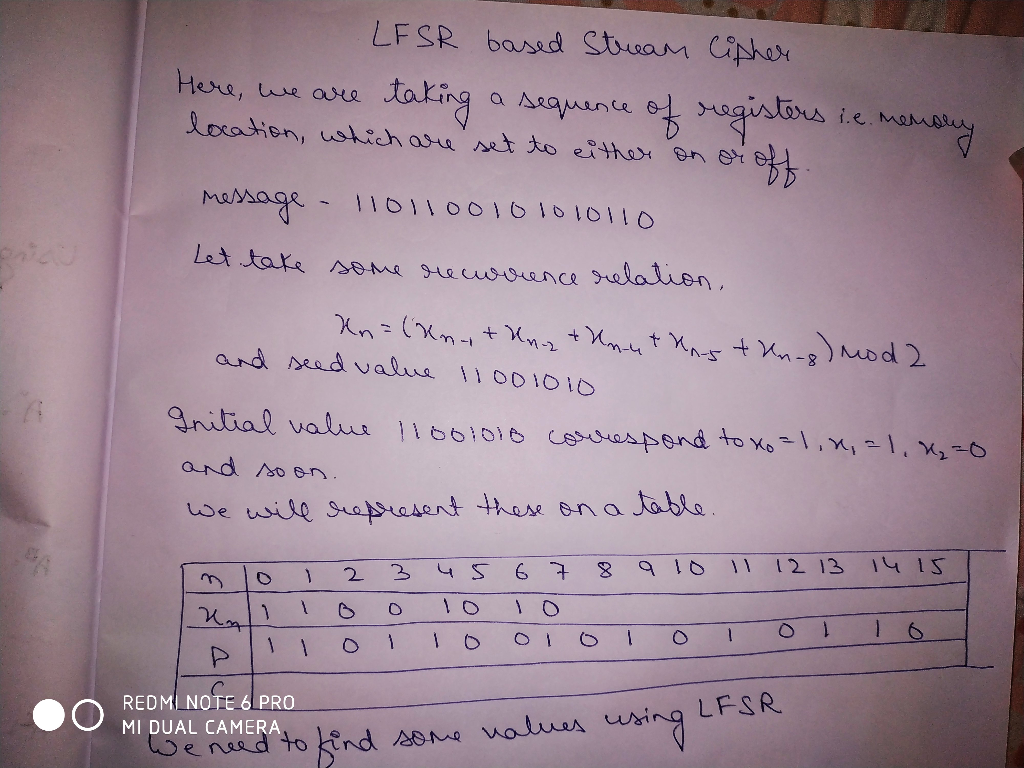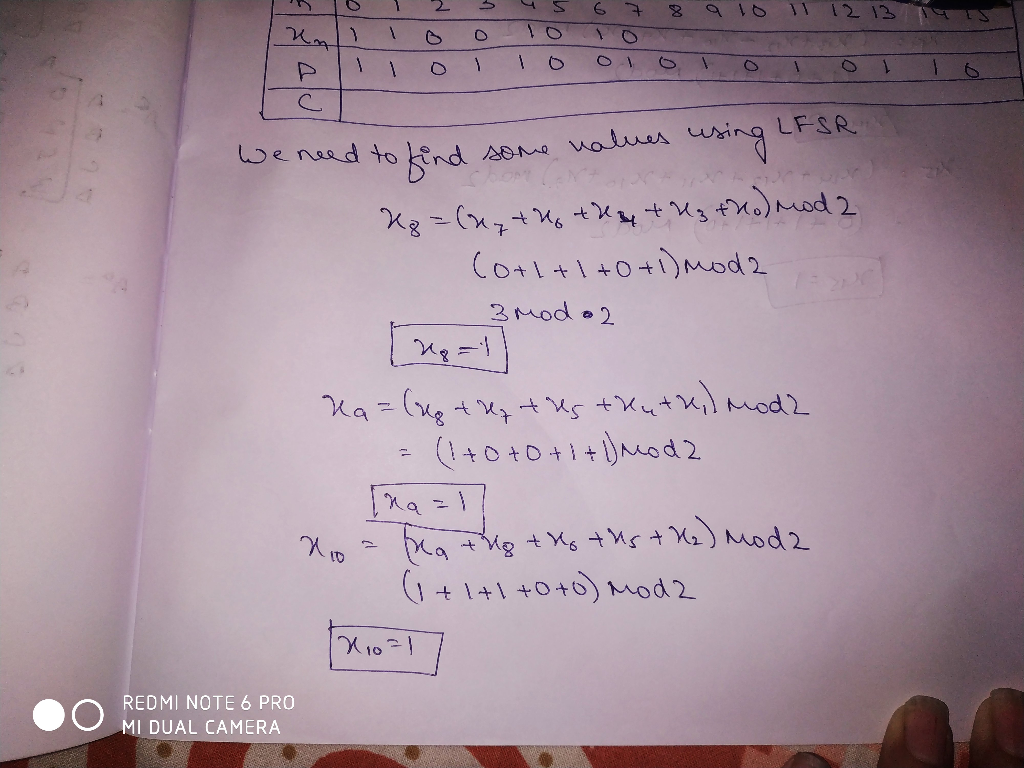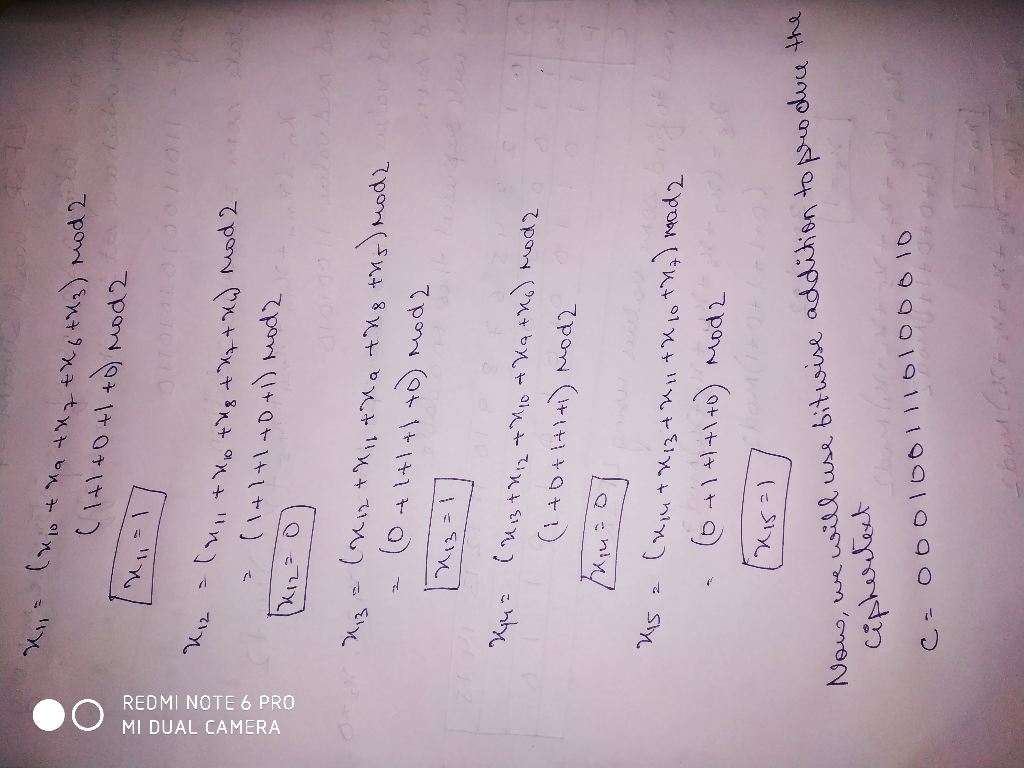##### Add Answer of: use LFSR to encrypt and decrypt some message, and use two or more different structure of LFSR.
Similar Homework Help Questions
• ### Develop an encryption and an decryption algorithm in Java, use two structure of LFSR, a complete...

Develop an encryption and an decryption algorithm in Java, use two structure of LFSR, a complete algorithm including main function to test .encrypt text data

• ### To encrypt or not to encrypt(decrypt)?

Program RequirementsYour program must meet the following requirements:1. You must ask the user if they want to perform an encryption or decryption operation.2. You must ask the user to enter the name of the file they want to encrypt or decrypt.3. You must get an encryption key from the user which can be up to 128 characters. The key must be all lower case alphabetic characters.4. You must have a function which takes the encryption key and creates an encryption...

• ### (d) Decrypt the ciphertext message LEWLYPLUJL PZ H NYLHA ALHJOLY that was encrypted with the shift cipher f(p) (p+7) mod 26. [10 points] (e) [Extra Credit - 5 points] Encrypt the message "BA"...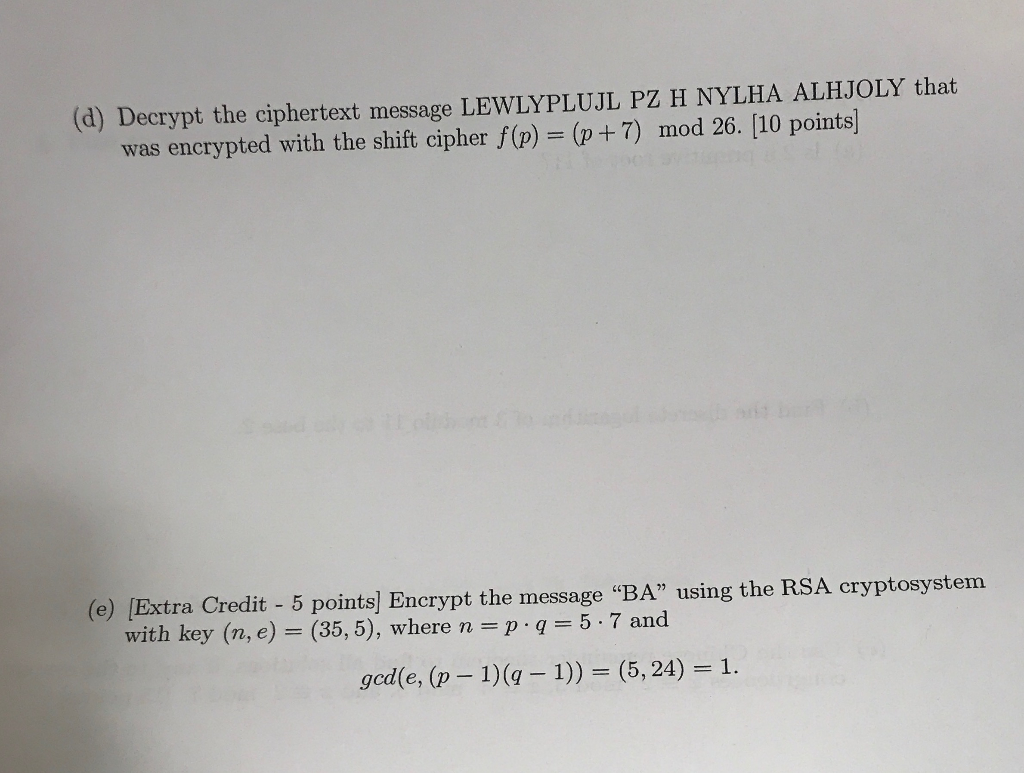(d) Decrypt the ciphertext message LEWLYPLUJL PZ H NYLHA ALHJOLY that was encrypted with the shift cipher f(p) (p+7) mod 26. [10 points] (e) [Extra Credit - 5 points] Encrypt the message "BA" using the RSA cryptosystem with key (ne) = (35,5), where n = p . q 5-7 and ged(e, (p-1) 1)) (5, 24) 1. 6. [5 points each (a) Is 2 a primitive root of 11? (b) Find the discrete logarithm of 3 modulo 11 to the base...

• ### C Programming - RSA encryption Hi there, I'm struggling with writing a C program to encrypt and decrypt a string usi...

C Programming - RSA encryption Hi there, I'm struggling with writing a C program to encrypt and decrypt a string using the RSA algorithm (C90 language only). It can contain ONLY the following libraries: stdio, stdlib, math and string. I want to use the following function prototypes to try and implement things: /*Check whether a given number is prime or not*/ int checkPrime(int n) /*to find gcd*/ int gcd(int a, int h) void Encrypt(); void Decrypt(); int getPublicKeys(); int getPrivateKeys();...

• ### RSA (Rivest–Shamir–Adleman) is an algorithm used by modern computers to encrypt and decrypt messages. It is an asymmetric cryptographic algorithm. Asymmetric means that there are two different keys. T...

RSA (Rivest–Shamir–Adleman) is an algorithm used by modern computers to encrypt and decrypt messages. It is an asymmetric cryptographic algorithm. Asymmetric means that there are two different keys. This is also called public key cryptography, because one of the keys can be given to anyone. The other key must be kept private. The algorithm is based on the fact that finding the factors of a large composite number is difficult: when the integers are prime numbers, the problem is called...

• ### An RSA cipher has public key pq = 65 and e = 7. Translate the message YES into its numeric equiv...

An RSA cipher has public key pq = 65 and e = 7. Translate the message YES into its numeric equivalent, and use the formula C = Me (mod pq) to encrypt the message. Decrypt the ciphertext 50 16 and translate the result into letters of the alphabet to discover the message.

• ### C Programming - RSA Encryption I've tried to write a program that can encrypt and decrypt strings using RSA and want...

C Programming - RSA Encryption I've tried to write a program that can encrypt and decrypt strings using RSA and want to be able to integrate it into a header file which contains codes for compression, linked list etc.. However, the use of global variables and fixed size of encryption is making it hard to do so Can someone please help me modify the following the code? I want to be able to just pass it in a string to...

• ### I need Help to Write a function in C that will Decrypt at least one word with a substitution cipher given cipher text an...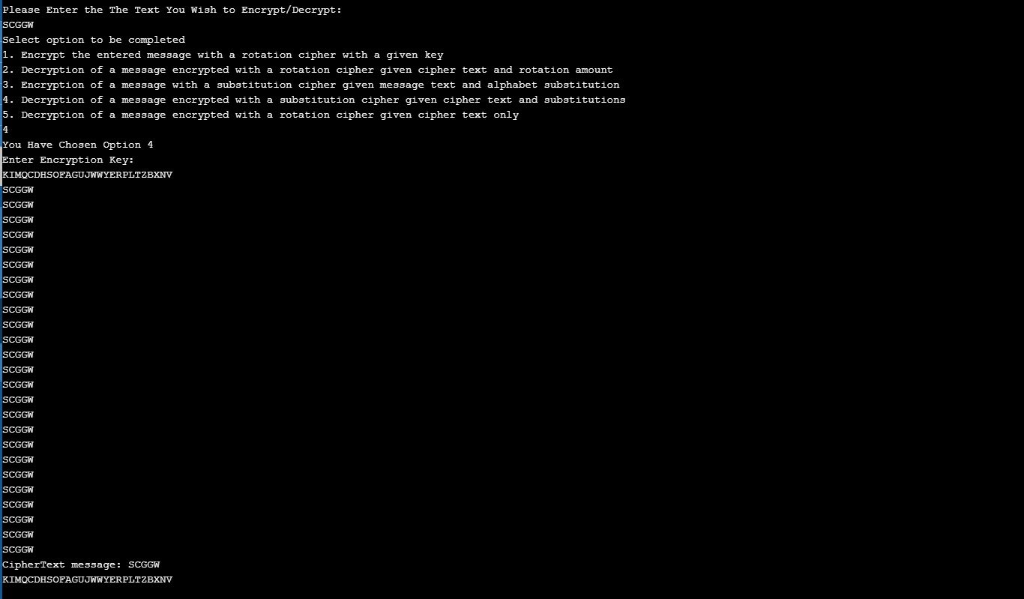I need Help to Write a function in C that will Decrypt at least one word with a substitution cipher given cipher text and key My Current Code is: void SubDecrypt(char *message, char *encryptKey) { int iteration; int iteration_Num_Two = 0; int letter; printf("Enter Encryption Key: \n");                                                           //Display the message to enter encryption key scanf("%s", encryptKey);                                                                   //Input the Encryption key for (iteration = 0; message[iteration] != '0'; iteration++)                               //loop will continue till message reaches to end { letter = message[iteration];                                                      ...

• ### 11. What sequence of pseudorandom numbers is generated using the linear congruential generator xn +1 (4xn + 1) mod 7 with seed Xo-37 12. Encrypt the message STOP POLLUTION by translating the letters...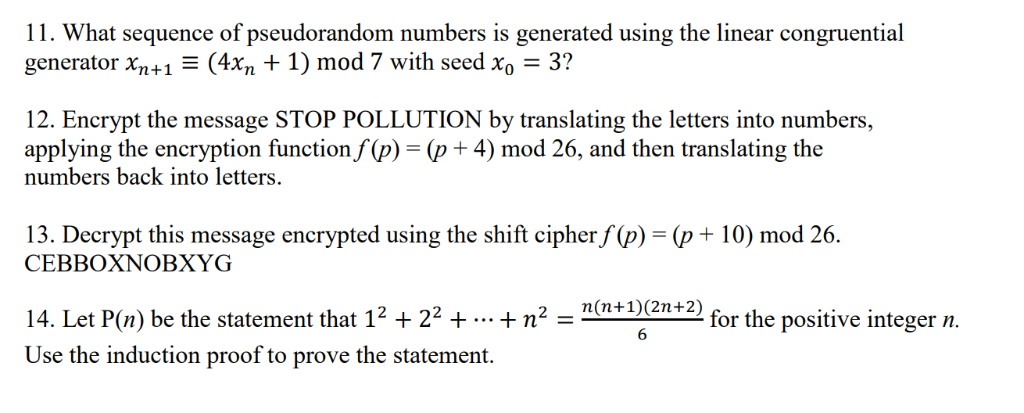11. What sequence of pseudorandom numbers is generated using the linear congruential generator xn +1 (4xn + 1) mod 7 with seed Xo-37 12. Encrypt the message STOP POLLUTION by translating the letters into numbers, applying the encryption function/ P)-(p + 4) mod 26, and then translating the numbers back into letters. 13. Decrypt this message encrypted using the shift cipher f (p) (p+ 10) mod 26 CEBBOXNOBXYG 14. Let P() be the statement that 12 +22 ++n2 -n-)(en+2) for...

• ### use java

Given input as a text file (.txt), encrypt and decrypt the text in the file using RSA encryption and decryption.Your program should prompt the user for the following input:1: Part 1 of RSA programming assignment 2: Part 2 of RSA programming assignment Please input "1" for part one and input "2" for part two ::Part 1:Input format:Your program should prompt the user for the following input:Enter the input file that you want to encrypt:Program functionality:The program should:? Select two primes...

Need Online Homework Help?